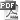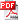#### Algorithmics, Programming (I1101)

The objective of this course is to introduce students to the basics of imperative programming which is one of the most effective paradigms of modern programming. Thus, the theme of algorithmics will be discussed to prepare the continuation of the course. Indeed, an algorithm expresses the logical structure of a solution to a given problem and this independently of any language and any machine. Algorithmics is one of the key steps in teaching programming. Then, it is a matter of applying the knowledge approached in algorithmics through the mastery of a programming language. This is precisely the purpose of this second part of this module which aims to equip students with the background necessary to master a given programming language, in this case the C language, which is at the base of many others. Our objectives are summarized as follows:

• Learn the basic concepts of algorithmics
• Be able to implement these concepts to analyze simple problems
• Mastering abstraction mechanisms in order to analyze a problem and systematically design correct and adequate algorithms and programs
• Implement, using the C language, the algorithms solving a particular problem
• Master the basics of modular programming

Chapter 0: Algorithmics
Chapter 1: Fundamental elements of a C programChapter 2: Basic types, operators and expressionsChapter 3: Read and write dataCompile and Run C Program using Visual Studio 2012Chapter 4: Alternative structureChapter 5: Repetitive structureChapter 6: ArraysChapter 7: StringsChapter 8: FunctionsTS 1TS 2TS *: Supplementary ExercisesTS 3TS 4